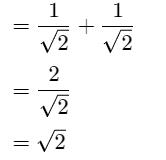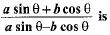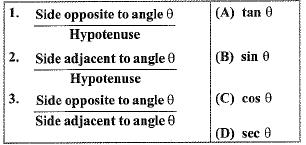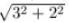Courses

# NTSE Level Test: Introduction To Trigonometry

## 10 Questions MCQ Test Mathematics (Maths) Class 10 | NTSE Level Test: Introduction To Trigonometry

Description
This mock test of NTSE Level Test: Introduction To Trigonometry for Class 10 helps you for every Class 10 entrance exam. This contains 10 Multiple Choice Questions for Class 10 NTSE Level Test: Introduction To Trigonometry (mcq) to study with solutions a complete question bank. The solved questions answers in this NTSE Level Test: Introduction To Trigonometry quiz give you a good mix of easy questions and tough questions. Class 10 students definitely take this NTSE Level Test: Introduction To Trigonometry exercise for a better result in the exam. You can find other NTSE Level Test: Introduction To Trigonometry extra questions, long questions & short questions for Class 10 on EduRev as well by searching above.
QUESTION: 1

### The value of (sin 30° + cos 30°) - (sin 60° + cos 60°) is

Solution:

sin 30° = 1/2,
cos 30°=√3/2,
sin 60°=√3/2,
cos 60°=1/2,
By putting the value of sin 30°, cos 30°, sin 60° and cos 60° in equation

We get=
(sin30°+cos30°)-(sin60°+cos60°)=(1/2+√3/2)-(√3/2+1/2)
=0

QUESTION: 2

### Ratios of sides of a right triangle with respect to its acute angles are known as

Solution:

The ratios of sides of a right-angled triangle with respect to any of its acute angles are known as the trigonometric ratios of that particular angle.

QUESTION: 3

### The value of (sin 45° + cos 45°) is

Solution:

sin 45° + cos 45°Hence, the answer is = √2

QUESTION: 4

If tan θ = a/b then the value ofSolution:

Let,angle= θ
(asinθ + bcosθ)/(asinθ - bcosθ)
Dividing both numerator and denominator from cosθ
We get,
atanθ +b/atanθ - b
= ( a.a/b + b) /(a.a/b - b) =(a²/b +b)/(a²/b - b)
=(a² + b²/a²- b²)

QUESTION: 5

If 6cotθ + 2cosecθ = cotθ + 5cosecθ, then cosθ is

Solution:

6cot+2cosec=cot+5cosec
6cot-cot=5cosec-2cosec
5cot=3cosec
5cos/sin=3/sin
cos=3/5

QUESTION: 6

Match the Columns:Solution:

Correct Answer :- b

Explanation : If θ is one of the acute angles in a triangle, then the sine of theta is the ratio of the opposite side to the hypotenuse, the cosine is the ratio of the adjacent side to the hypotenuse, and the tangent is the ratio of the opposite side to the adjacent side.

QUESTION: 7

9 sec2 A - 9tan2 A is equal to

Solution:

9 sec2 A - 9 tan2 A
= 9( sec2 A - tan2 A)
= 9 × 1
= 9

QUESTION: 8

The value of sin2 30° - cos2 30° is

Solution:

Solution :- sin^2 30° − cos^2 30°

= (1/2)2 −((3)1/2/2)2

= 1/4 - 3/4

= -1/2

QUESTION: 9

If tan A = 3/2, then the value of cos A is

Solution:

Tanθ = Perpendicular / Base
We are given that TanA = 3/2
On comparing
Perpendicular = 3
Base = 2
To fing hypotenuse
Hypotenuse2 = Perpendicular2 + Base2
Hypotenuse2 = 32 + 22
Hypotenuse =Hypotenuse = 3.6

Cosθ = Base / Hypotenuse
CosA = 2 / 3.6
Hence the value of Cos A is 2/3.6=2/√13

QUESTION: 10

If 3 cot θ = 2, then the value of tan θ

Solution:

3 cot θ = 2 ⇒ cot θ = 2/3 ⇒ tan θ = 3/2